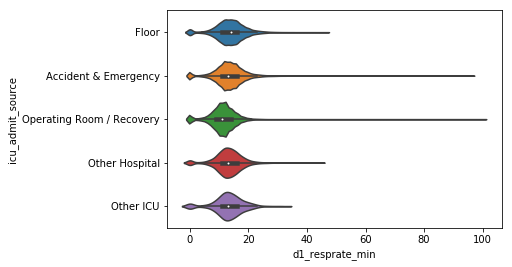### Categorical Data :

The two main categories of categorical data are nominal and ordinal.

In nominal categorical data attribute, there is no concept of ordering amongst the values of that attribute.

Ordinal categorical attributes have some sense or notion of order amongst its values.

The common plots used to visualize categorical data are

**1.Count Plot
2.Bar Plot
3.Box Plot
4.Violin Plot **

In :
``````import numpy as np
import pandas as pd # data processing, CSV file I/O (e.g. pd.read_csv)
import seaborn as sns
import matplotlib.pyplot as plt

%matplotlib inline
``````
In :
``````# loading dataset
df = pd.read_csv("../input/widsdatathon2020/training_v2.csv")``````
In :
``print([c for c in df.columns if (1<df[c].nunique()) & (df[c].dtype != np.number)& (df[c].dtype != int) ])``
```['ethnicity', 'gender', 'hospital_admit_source', 'icu_admit_source', 'icu_stay_type', 'icu_type', 'apache_3j_bodysystem', 'apache_2_bodysystem'] ```
In :
``````categorical_cols =  ['hospital_id',
'ethnicity', 'gender', 'hospital_admit_source', 'icu_admit_source', 'icu_stay_type', 'icu_type', 'apache_3j_bodysystem', 'apache_2_bodysystem',"hospital_death","d1_heartrate_min" ,"d1_lactate_min","d1_resprate_min"]``````
In :
``````Categorical_df= df[categorical_cols]
Categorical_df.head(5)``````
Out:

### Countplot :

A count plot can be thought of as a histogram across a categorical, instead of quantitative, variable. The basic API and options are identical to those for barplot(), so you can compare counts across nested variables.

In :
``sns.countplot(data = Categorical_df, x = 'gender',hue ='hospital_death')``
Out:
``<matplotlib.axes._subplots.AxesSubplot at 0x7fbf4cb465c0>``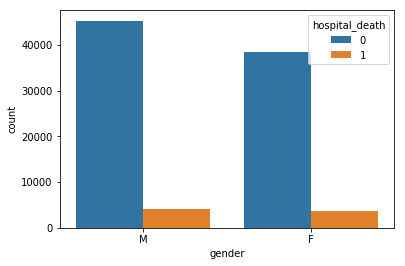In :
``sns.countplot(y="ethnicity", hue="hospital_death" , data=Categorical_df);``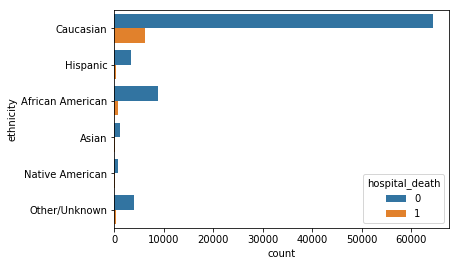### BarPlot :

A bar plot represents an estimate of central tendency for a numeric variable with the height of each rectangle and provides some indication of the uncertainty around that estimate using error bars.

In :
``sns.barplot(y="apache_3j_bodysystem", x="hospital_death" , data=Categorical_df);``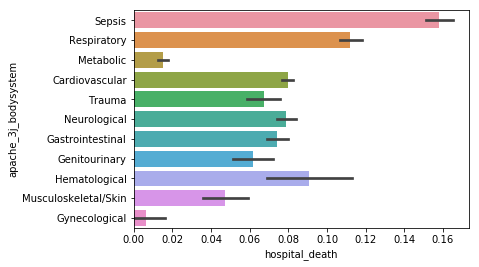### Boxplot :

A boxplot is a standardized way of displaying the distribution of data based on a five number summary (“minimum”, first quartile (Q1), median, third quartile (Q3), and “maximum”).

In :
``sns.boxplot(x="d1_heartrate_min", y="apache_3j_bodysystem" , data=Categorical_df);``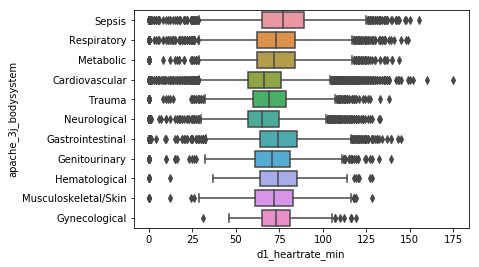### ViolinPlot:

A violin plot plays a similar role as a box and whisker plot. It shows the distribution of quantitative data across several levels of one (or more) categorical variables such that those distributions can be compared.

In :
``sns.violinplot(x="d1_resprate_min", y="icu_admit_source" , data=Categorical_df);``